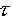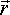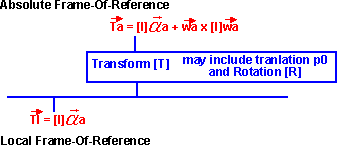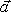# Physics - Rotation - Changing Frame-Of-Reference

At first, it may seem a bit academic to transform these quantities into different frames-of-reference but, this is important for solving practical problems like collisions. For instance, the two objects colliding will have their inertial tensors defined in their own local coordinates, but when we work out the collision response, the impulse will have to be calculated in some common coordinate system.What we are doing here is observing the same particle or solid object from different frames-of-reference. When the frames-of-reference are static (not moving relative to each other). Then the Newtonian laws will apply, regardless of where, or which direction, that we are looking at them from, provided that we are consistent about measuring all quantities on the same frame-of-reference.

It may be that the transform is changing with time, for example, if we are trying to solve a collision response, we might want to work in the frame-of-reference of one of the objects that is colliding. This object may be moving, so its frame-of-reference is moving with respect to the absolute coordinate system.

Do all the laws of physics apply when observing them from a moving frame-of-reference? If the frame-of-reference is moving with a constant linear velocity, then the Newtonian laws will apply just the same. A stationery object in one frame-of-reference may appear to be moving in another, but provided that we are constant about which frame-of-reference that we are working in, neither will contravene the laws. (Einsteins laws may not apply, the speed of light is the same in each frame-of-reference, relativity is not relative to the frame-of-reference - we are interested in slow speed interactions so this is not an issue for us).

However, if the frame-of-reference has angular motion (even if its constant), or if the frame-of-reference is accelerating, or if it has some irregular motion, then the Newtonian laws will not apply in this frame-of-reference.

To take an example, imagine a solid object travailing in free space, it may be spinning and have linear velocity as well. We may want to work in this objects own coordinate system, because this frame-of-reference is rotating, the Newtonian laws may not apply, for example an object which is stationary in the absolute frame-of-reference will appear to be traveling in a spiral in this objects frame of reference. An object with no forces acting on it should not be moving in a spiral, so Newtonian laws do not apply in this frame-of-reference. However, if we want to apply this inertia tensor, then we have to work in the local coordinate system of the rotating object.xyz
=
 ixx ixy ixz iyx iyy iyz izx izy izzxyz

So, we have to be careful. When we are calculating torques and forces, we often want to work in its local coordinate system, but when we are calculating motion we probably want to work in absolute coordinates.

### Using matrix algebra to calculate transforms to other frames-of-reference

As described here, a 4x4 matrix can be used to represent a rotation and a translation in 3 dimensions. So we can use matrix algebra to translate from one frame of reference to another.

The transform will take a 3d vector representing a point in absolute coordinates and convert it into a 3d vector representing a point in absolute coordinates:a = [T]l

It is possible to have many layers of one frames-of-reference inside another. For example, if we know the position of the moon relative to the earth [Tme] and we know the position of the earth in the frame-of-reference of the sun [Tes], then we could work out the position of a point on the moon in the frame of reference of the sun [Tms].

It turns out that transforms can be concatenated by multiplying their corresponding matrices. So [TMs] = [Tes]*[Tme].

One way to represent this is to use a scene graph. We could use a scene graph is a similar way to VRML. We could put dynamics information into the scene graph in the same way that shape information is. In this page I would like to work out the effect of transforming dynamics information in this way.The transform allows us to plug in a coordinate in the local frame-of-reference and get the coresponding coordinate in the absolute frame-of-reference.

 Pax Pay Paz 1
=
 rxx rxy rxz tx ryz ryy ryz ty rzx rzy rzz tz 0 0 0 1
 Plx Ply Plz 1
=
 Plx * rxx + Ply * rxy + Plz * rxz + tx Plx * ryz + Ply * ryy + Plz * ryz + ty Plx * rzx + Ply * rzy + Plz * rzz + tz 1

For Solid objects, we need to specify both the location and the orientation of the object to define its position. So for solid objects we can easily translate its centre-of-mass (c-of-m) in this way, but how do we translate its orientation? One way that occurred to me would be to, take a point an infinitesimal distance away from from the c-of-m, translate that, and then see the orientation of this point relative to the translated c-of-m.

 Pax' Pay' Paz' 1
=
 rxx rxy rxz tx ryx ryy ryz ty rzx rzy rzz tz 0 0 0 1
 Plx + dPlx Ply + dPly Plz + dPlz 1
=
 (Plx + dPlx) * rxx + (Ply + dPly) * rxy + (Plz + dPlz) * rxz + tx (Plx + dPlx) * ryz + (Ply + dPly) * ryy + (Plz + dPlz) * ryz + ty (Plx + dPlx) * rzx + (Ply + dPly) * rzy + (Plz + dPlz) * rzz + tz 1

So the relative position is given by:

 dPax dPay dPaz 1
=
 Pax' Pay' Paz' 1
-
 Pax Pay Paz 1
=
 dPlx * rxx + dPly * rxy + dPlz * rxz dPlx * ryz + dPly * ryy + dPlz * ryz dPlx * rzx + dPly * rzy + dPlz * rzz 1
=
 rxx rxy rxz 0 ryx ryy ryz 0 rzx rzy rzz 0 0 0 0 1
 dPlx dPly dPlz 1

So to transform a movement, then we transform it by the rotational part of the transform without the translational part of the matrix. Alternatively, if we don't want to modify the transform matrix, we could just use 0 for the 4th row of a relative movement vector, then the translational part will automatically be ignored.

 dPax dPay dPaz 0
= [T]
 dPlx dPly dPlz 0

But if we measuring orientation with 3 angles, θx, θy and θz. How can we translate this with [T]? This may seem a bit stange as we are using [T] to define the rotation of the frame-of-reference and θx, θy and θz to define the orientation in each frame-of-reference. The advantage of using θx, θy and θz would be that this notation would fit in better with the physics equations.

### Using 6 dimensional vectors to calculate transforms to other frames of reference

For Solid objects, we need to specify both the location and the orientation of the object to define its position. It might be useful if we could represent the transform of both location and orientation in one equation. To do this we need a 6x6 matrix as the position has 6 degrees of freedom.

I am not sure if this will work for position because the normal rules of algebra don't apply when combining rotations (see rotations). Can anyone help me prove if this will work?

 θax θay θaz pax pay paz
=
 m00 m01 m02 m03 m04 m05 m10 m11 m12 m13 m14 m15 m20 m21 m22 m23 m24 m25 m30 m31 m32 m33 m34 m35 m40 m41 m42 m43 m44 m45 m50 m51 m52 m53 m54 m55
 θlx θly θlz plx ply plz

However I am sure it would work for velocities because infinitesimally small rotations can be combined in the usual way.

 wax way waz vax vay vaz
=
 m00 m01 m02 m03 m04 m05 m10 m11 m12 m13 m14 m15 m20 m21 m22 m23 m24 m25 m30 m31 m32 m33 m34 m35 m40 m41 m42 m43 m44 m45 m50 m51 m52 m53 m54 m55
 wx wy wz vx vy vz

This 6x6 matrix does not contain any extra information than the 4x4 transform [T] (even the 4x4 transform has redundant information when we are considering only a rotation and translation).

However, multiplication of a 6d vector by a 6x6 matrix, might be a lot easier than multiplying 4x4 matrix by a 4x4 matrix (which we would have to do several times if we are going to represent rotations by using matrices).

The advantage of this notation is that:

• The linear and angular quantities are dealt with is the same equation.
• The angular velocities and accelerations are defined in a way that is consistent with the usual dynamics equations.

Issues:

• Not sure if we can use this to transform points.
• Not sure how we would concatinate transforms (transform within a transform).

## Point ()

Every coordinate point in the local frame of reference can be transformed to a coordinate in the absolute frame of referencea = [T]l . This can be thought of as the same point just measured in a different coordinate reference. A solid object can be transformed in this way by seperately transforming each point that makes it up.This transform [T] is a 4x4 matrix and is capable of representing lots of tranforms such as sheer, scaleing, reflection, etc. which are not valid operations for a solid body, also it is very wasteful using a 4x4 matrix. Since we only want to represent translations and rotations, it may be more efficient to represent it in terms of a 3x3 rotation matrix [R] and a translation0.a = [R]l +0

Where:

•a is the position in absolute coordinates.
• [R] is a 3x3 rotational matrix
•l is the position in local coordinates.
•0 is the linear translation.

But we still have the problem that [R] could be used to repesent invalid operations for a solid object, we need to ensure that [R] is orthogonal. The problem is that if a calculation involves lots of transforms then rounding errors could distort [R], therefore we need to normalise (or should that be orthogonalise) after tranforms are combined.

One way round this would be to work from the quaternion [Q] to represent the rotation, as the quaternion is easier to normalise:a =
 1 - 2 * ( yy + zz ) 2 * ( xy - zw ) 2 * ( xz + yw ) 2 * ( xy + zw ) 1 - 2 * ( xx + zz ) 2 * ( yz - xw ) 2 * ( xz - yw ) 2 * ( yz + xw ) 1 - 2 * ( xx + yy )l +0

where:

• xx = qx* qx
• xy = qx * qy
• xz = qx * qz
• xw = qx * qw
• yy = qy * qy
• yz = qy * qz
• yw = qy * qw
• zz = qz * qz
• zw = qz * qw
• qx, qy, qz and qw are the values of the quaternion.

## Linear Velocity()

As covered in kinematics the absolute velocity of a point is the velocity in the local coordinates plus the velocity of the frame of reference.a =r +i

## Angular Velocity ()

As covered in kinematics the absolute angular velocity of a solid object is the angular velocity in the local coordinates plus the angular velocity of the frame of reference.a =r +i

## Angular Momentum ()

The angular momentum about any pointis the angular momentum about the centre of mass, plus the angular momentum associated with the motion of the centre of mass about. Is this true for the most general case? Can anyone help me prove this?

In the local frame-of-reference then the angular momentum is:l =l xll =l x ml

where:

•l = Angular momentum in local frame-of-reference.
•l = distance of particle from centre of rotation.
•l = linear momentum in local frame-of-reference.
• m = mass of particle
•l =linear velocity in local frame-of-reference.

In the absolute frame-of-reference then the angular momentum is:a =a xaa =a x ma

where:

•a = Angular momentum in absolute frame-of-reference.
•a = distance of particle from centre of rotation.
•a = linear momentum in absolute frame-of-reference.
• m = mass of particle
•a =linear velocity in absolute frame-of-reference.

## Angular Acceleration ()

Can anyone help me derive the angular acceleration in absolute coordinates, given the angular acceleration in local coordinates and the motion of the local frame-of-reference?

## Torque ()In the local frame-of-reference (the inertial frame) then we have shown here that:l = [I]a

Where:

•l is the instantaneous torque about PC as seen in the local coordinates
• [I] is a 3x3 matrix, known as the inertial tensor matrix.
•a Is the instantaneous angular acceleration about PC

In the absolute frame-of-reference then the following equation applies, this is known as eulers equation:a = [I]a + wa x [I]wa

Where:

•a is the instantaneous torque about PC
• [I] is a 3x3 matrix, known as the inertial tensor matrix.
•a Is the instantaneous angular acceleration about PC
• wa Is the instantaneous angular velocity about PC

So when we move out of the inertial frame there is an apparent change in torque of wa x [I]wa which is just due to the rotating viewpoint.

Can anyone help me to derive eulers equation so that I can understand what assumptions that it makes?

Am I correct that [I] is the same in both of these equations? ie the inertial tensor defined in the inertial frame.

Is it possible to solve the more general case, that is where an object has a torque applied in the local frame, but the frame itself is rotating in a competely different axis? In other words there is a hierarchy of rotating transoforms. This would allow us to solve gyrosope type problems, such as when a man is holding a spinning wheel at arms length, and is standing on a rotating platform, and attempts to lift the spinning wheel.

## Inertia [I]

I think the inertial tensor defined in the inertial frame is always used as this has constant terms. Although I guess it would be possible to define an inertial tensor such that:a = [I(t)]a

provided that we were prepared to accept that the terms of [I(t)] will be a function of time.

 metadata block see also: Correspondence about this page Book Shop - Further reading. Where I can, I have put links to Amazon for books that are relevant to the subject, click on the appropriate country flag to get more details of the book or to buy it from them.Engineering Mechanics Vol 2: Dynamics - Gives theory for rigid dynamics, aims to allow prediction of effects of force and motion. Includes rotating frame of reference. Lots of colour diagrams, I guess its college / University level. Commercial Software Shop Where I can, I have put links to Amazon for commercial software, not directly related to the software project, but related to the subject being discussed, click on the appropriate country flag to get more details of the software or to buy it from them.

This site may have errors. Don't use for critical systems.